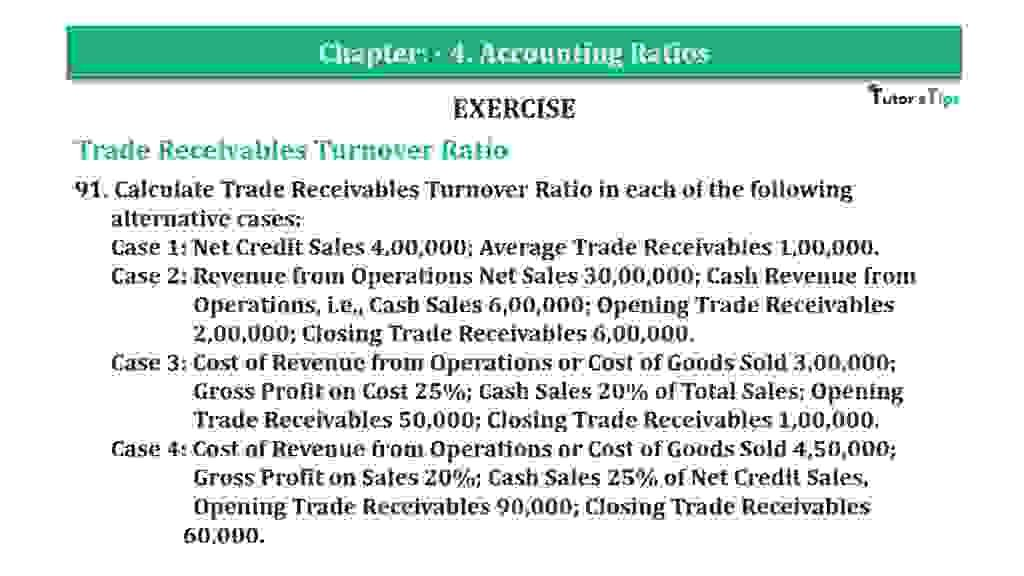# Question 91 Chapter 4 of +2-B – T.S. Grewal 12 ClassQuestion 91 Chapter 4 of +2-B

Trade Receivables Turnover Ratio

91. Calculate Trade Receivables Turnover Ratio in each of the following
alternative cases:
Case 1: Net Credit Sales 4,00,000; Average Trade Receivables 1,00,000.
Case 2: Revenue from Operations Net Sales 30,00,000; Cash Revenue from
Operations, i.e., Cash Sales 6,00,000; Opening Trade Receivables
2,00,000; Closing Trade Receivables 6,00,000.
Case 3: Cost of Revenue from Operations or Cost of Goods Sold 3,00,000;
Gross Profit on Cost 25%; Cash Sales 20% of Total Sales; Opening
Trade Receivables 50,000; Closing Trade Receivables 1,00,000.
Case 4: Cost of Revenue from Operations or Cost of Goods Sold 4,50,000;
Gross Profit on Sales 20%; Cash Sales 25% of Net Credit Sales,
Opening Trade Receivables 90,000; Closing Trade Receivables 60,000.

### The solution of Question 91 Chapter 4 of +2-B: –

Case I :

 Trade Receivables Turnover Ratio = Net Credit Sales Average Trade Receivable
 3 = Rs. 4,00,000 Rs. 1,00,000 = 4 Times

Case II :

 Net Credit Sales = Total Sales −Cash Sales = Rs. 30,00,000 – Rs. 6,00,000 = Rs. 24,00,000
 Average Trade Receivable = Opening Trade Receivable + Closing Trade Receivable 2
 = Rs. 2,00,000 + Rs. 6,00,000 2 Trade Receivables Turnover Ratio = Net Credit Sales Average Trade Receivable = Rs. 24,00,000 Rs. 4,00,000 = 6 Times

Case III :

 Cost of Goods Sold = Rs. 3,00,000 Gross Profit = 25% on Cost Gross Profit = 25 XRs.3,00,000 100 Rs. 75,000 = Cost of Goods Sold + Gross Profit = Rs. 3,00,000 + Rs. 75,000 = Rs. 3,75,000 Cash Sales = 20% of Total Sales Gross Profit = 25% on Cost Cash Sales = 20 XRs.3,75,000 100 = Rs. 75,000 Credit Sales = Total Sales − Cash Sales = Rs. 3,75,000 – Rs. 75,000 = Rs. 3,00,000

 Average Trade Receivable = Opening Trade Receivable + Closing Trade Receivable 2 = Rs. 50,000 + Rs. 1,00,000 2 = Rs. 75,000 Trade Receivables Turnover Ratio = Net Credit Sales Average Trade Receivable X = Rs. 3,00,000 Rs. 75,000 = 4 Times

Case IV

Sales = X

 Cash Sales = X x 20 100 = 20x 100

Sales = Cost of goods Sold + Gross Profit

 X = 4,50,000 + 20x 100
 X x 100 = Rs. 4,50,000 x 100 + 20x 100x = Rs. 4,50,00,000 + 20x 100x – 20x = Rs. 4,50,00,000 80x = Rs. 4,50,00,000
 X = Rs. 4,50,00,000 80 = Rs. 5,62,000 Credit Sales = Y

 Cash Sales = Y x 20 100 = 20y 100

Sales = Cash Sales + Credit Sales

 Rs. 5,62,000 = 25y +y 100
 Rs. 5,62,000 = 25y 100 Rs. 5,62,500X 100 = 125y 125y = Rs.5,62,50,000
 = Rs. 5,62,50,000 125 = Rs. 4,50,000
 Average Trade Receivable = Opening Trade Receivable + Closing Trade Receivable 2 = Rs. 90,000 + Rs. 60,000 2 = Rs. 75,000 Trade Receivables Turnover Ratio = Net Credit Sales Average Trade Receivable X = Rs. 4,50,000 Rs. 75,000 = 4 Times

Balance Sheet: Meaning, Format & Examples

Thanks, Please Like and share with your friends

Comment if you have any question.

Also, Check out the solved question of previous Chapters: –

### T.S. Grewal’s Double Entry Book Keeping (Vol. II: Accounting for Companies)T.S. Grewal’s Analysis of Financial Statements